# A polynomial-time algorithm for determining quadratic Lyapunov functions for nonlinear systems

L. Vandenberghe and S. Boyd

Proceedings of the European Conference on Circuit Theory and Design, pages 1065–1068, 1993.

We consider nonlinear systems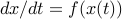where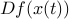is known to lie in the convex hull of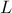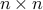matrices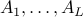. For such systems, quadratic Lyapunov functions can be determined using convex programming techniques. This paper describes an algorithm that either finds a quadratic Lyapunov function or terminates with a proof that no quadratic Lyapunov function exists. The algorithm is an interior-point method based on the theory developed by Nesterov and Nemirovsky.